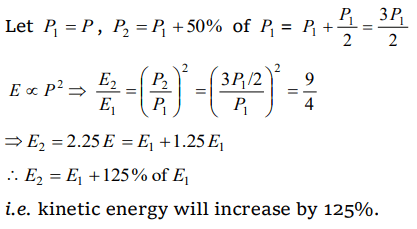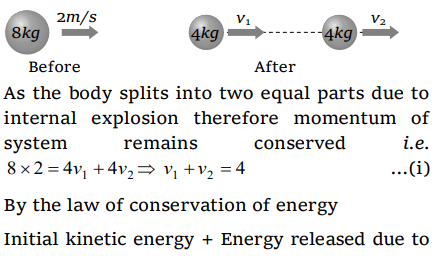## Work, Energy and Power Questions and Answers Part-8

1. The bob of a simple pendulum (mass m and length l) dropped from a horizontal position strikes a block of the same mass elastically placed on a horizontal frictionless table. The K.E. of the block will be
a) 2 mgl
b) mgl/2
c) mgl
d) 0

Explanation:2. From a stationary tank of mass 125000 pound a small shell of mass 25 pound is fired with a muzzle velocity of 1000 ft/sec. The tank recoils with a velocity of
a) 0.1 ft/sec
b) 0.2 ft/sec
c) 0.4 ft/sec
d) 0.8 ft/sec

Explanation: According to conservation of momentum
Momentum of tank = Momentum of shell
125000 × vtank = 25 × 1000
vtank = 0.2 ft/sec

3. A bomb of 12 kg explodes into two pieces of masses 4 kg and 8 kg. The velocity of 8kg mass is 6 m/sec. The kinetic energy of the other mass is
a) 48 J
b) 32 J
c) 24 J
d) 288 J

Explanation: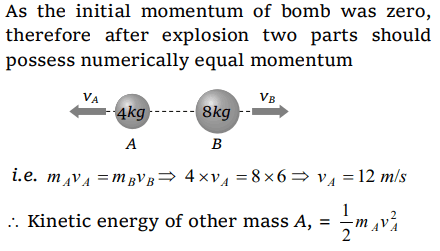4. A rifle bullet loses $1\diagup20^{th}$ of its velocity in passing through a plank. The least number of such planks required just to stop the bullet is
a) 5
b) 10
c) 11
d) 20

Explanation: Let the thickness of one plank is s
if bullet enters with velocity u then it leaves with velocity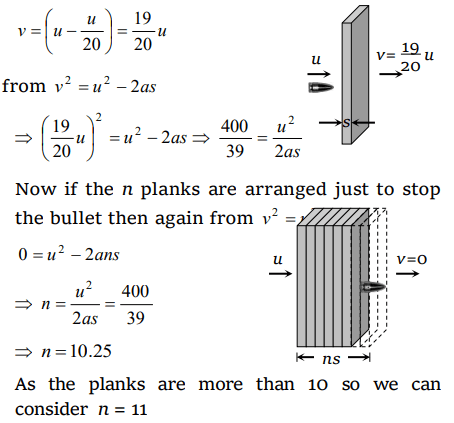5. A body of mass 2 kg is thrown up vertically with K.E. of 490 joules. If the acceleration due to gravity is 9.8 $m\diagup s^{2}$ , then the height at which the K.E. of the body becomes half its original value is given by
a) 50 m
b) 12.5 m
c) 25 m
d) 10 m

Explanation: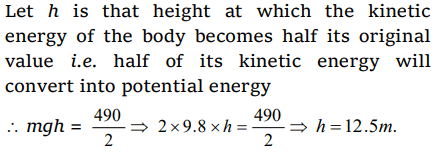6. Two masses of 1 gm and 4 gm are moving with equal kinetic energies. The ratio of the magnitudes of their linear momenta is
a) 4 : 1
b) $\sqrt{2}:1$
c) 1 : 2
d) 1 : 16

Explanation: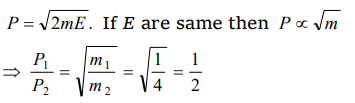7. If the K.E. of a body is increased by 300%, its momentum will increase by
a) 100%
b) 150%
c) $\sqrt{300}%$
d) 175%

Explanation: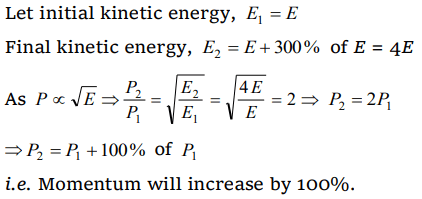8. A light and a heavy body have equal kinetic energy. Which one has a greater momentum ?
a) The light body
b) The heavy body
c) Both have equal momentum
d) It is not possible to say anything without additional information

Explanation: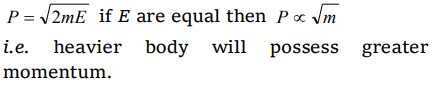9. If the linear momentum is increased by 50%, the kinetic energy will increase by
a) 50%
b) 100%
c) 125%
d) 25%